Courses

# Direct Electric Current Physics Notes | EduRev

## Physics : Direct Electric Current Physics Notes | EduRev

The document Direct Electric Current Physics Notes | EduRev is a part of the Physics Course Basic Physics for IIT JAM.
All you need of Physics at this link: Physics

Introduction:

Direct current, abbreviation DC, flow of electric charge that does not change direction. Direct current is produced by batteries, fuel cells, rectifiers, and generators with commutators. Direct current was supplanted by alternating current (AC) for common commercial power in the late 1880s because it was then uneconomical to transform it to the high voltages needed for long-distance transmission. Techniques that were developed in the 1960s overcame this obstacle, and direct current is now transmitted over very long distances, even though it must ordinarily be converted to alternating current for final distribution. For some uses, such as electroplating, direct current is essential. See also electric current.

Basic Phenomena and Principles:

Many electric phenomena occur under what is termed steady-state conditions. This means that such electric quantities as current, voltage, and charge distributions are not affected by the passage of time. For instance, because the current through a filament inside a car headlight does not change with time, the brightness of the headlight remains constant. An example of a nonsteady-state situation is the flow of charge between two conductors that are connected by a thin conducting wire and that initially have an equal but opposite charge. As current flows from the positively charged conductor to the negatively charged one, the charges on both conductors decrease with time, as does the potential difference between the conductors. The current therefore also decreases with time and eventually ceases when the conductors are discharged.
In an electric circuit under steady-state conditions, the flow of charge does not change with time and the charge distribution stays the same. Since charge flows from one location to another, there must be some mechanism to keep the charge distribution constant. In turn, the values of the electric potentials remain unaltered with time. Any device capable of keeping the potentials of electrodes unchanged as charge flows from one electrode to another is called a source of electromotive force, or simply an emf.
Figure 12 shows a wire made of a conducting material such as copper. By some external means, an electric field is established inside the wire in a direction along its length. The electrons that are free to move will gain some speed. Since they have a negative charge, they move in the direction opposite that of the electric field. The current i is defined to have a positive value in the direction of flow of positive charges. If the moving charges that constitute the current i in a wire are electrons, the current is a positive number when it is in a direction opposite to the motion of the negatively charged electrons. (If the direction of motion of the electrons were also chosen to be the direction of a current, the current would have a negative value.) The current is the amount of charge crossing a plane transverse to the wire per unit time—i.e., in a period of one second. If there are n free particles of charge q per unit volume with average velocity v and the cross-sectional area of the wire is A, the current i, in elementary calculus notation, is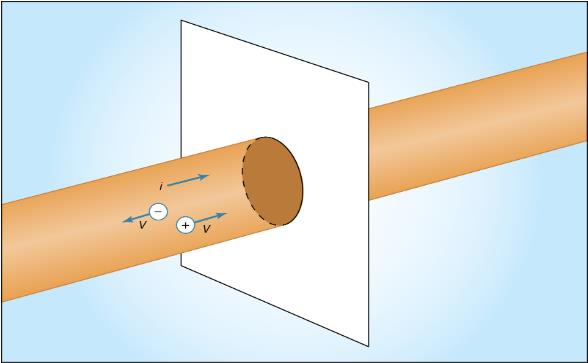Figure 12: Motion of charge in electric current i (see text).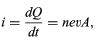(15)

where dQ is the amount of charge that crosses the plane in a time interval dt. The unit of current is the ampere (A); one ampere equals one coulomb per second. A useful quantity related to the flow of charge is current density, the flow of current per unit area. Symbolized by J, it has a magnitude of i/A and is measured in amperes per square metre.
Wires of different materials have different current densities for a given value of the electric field E; for many materials, the current density is directly proportional to the electric field. This behaviour is represented by Ohm’s law: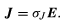(16)

The proportionality constant σJ is the conductivity of the material. In a metallic conductor, the charge carriers are electrons and, under the influence of an external electric field, they acquire some average drift velocity in the direction opposite the field. In conductors of this variety, the drift velocity is limited by collisions, which heat the conductor.

If the wire in Figure 12 has a length l and area A and if an electric potential difference of V is maintained between the ends of the wire, a current i will flow in the wire. The electric field E in the wire has a magnitude V/l. The equation for the current, using Ohm’s law, is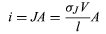(17)

Or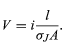(18)

The quantity lJA, which depends on both the shape and material of the wire, is called the resistance R of the wire. Resistance is measured in ohms(Ω). The equation for resistance,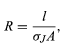(19)

is often written as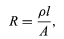(20)

where ρ is the resistivity of the material and is simply 1/σJ. The geometric aspects of resistance in equation (20) are easy to appreciate: the longer the wire, the greater the resistance to the flow of charge. A greater cross-sectional area results in a smaller resistance to the flow.(20)

The resistive strain gauge is an important application of equation (20). Strain, δl/l, is the fractional change in the length of a body under stress, where δl is the change of length and l is the length. The strain gaugeconsists of a thin wire or narrow strip of a metallic conductor such as constantan, an alloy of nickel and copper. A strain changes the resistance because the length, area, and resistivity of the conductor change. In constantan, the fractional change in resistance δR/R is directly proportional to the strain with a proportionality constant of approximately 2.
A common form of Ohm’s law is

V=iR   (21)

where V is the potential difference in volts between the two ends of an element with an electric resistance of R ohms and where i is the current through that element.
Table 2 lists the resistivities of certain materials at room temperature. These values depend to some extent on temperature; therefore, in applications where the temperature is very different from room temperature, the proper values of resistivities must be used to calculate the resistance. As an example, equation (20) shows that a copper wire 59 metres long and with a cross-sectional area of one square millimetre has an electric resistance of one ohm at room temperature.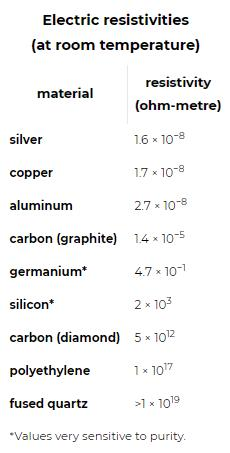Direct-Current Circuits:
The simplest direct-current (DC) circuit consists of a resistor connected across a source of electromotive force. The symbol for a resistor is shown in Figure 15; here the value of R, 60Ω, is given by the numerical value adjacent to the symbol. The symbol for a source of electromotive force, E, is shown with the associated value of the voltage. Convention gives the terminal with the long line a higher (i.e., more positive) potential than the terminal with the short line. Straight lines connecting various elements in a circuit are assumed to have negligible resistance, so that there is no change in potential across these connections. The circuit shows a 12-volt electromotive force connected to a 60Ω resistor. The letters a, b, c, and d on the diagram are reference points.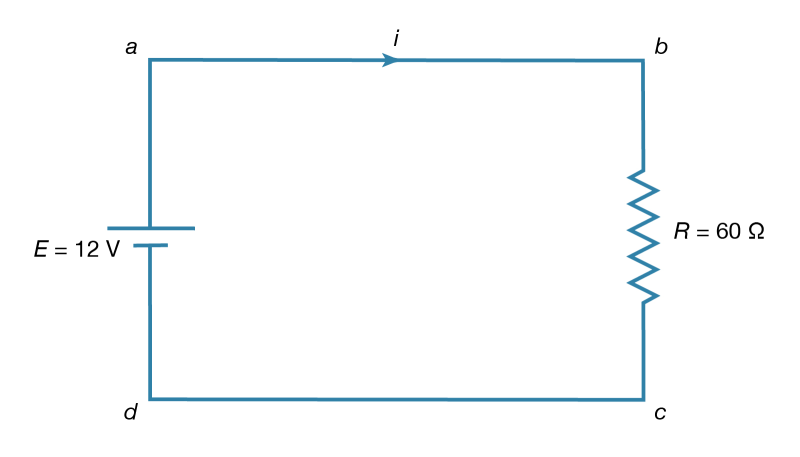Figure 15: Direct-current circuit (see text).

The function of the source of electromotive force is to maintain point a at a potential 12 volts more positive than point d. Thus, the potential difference Va − Vd is 12 volts. The potential difference across the resistance is Vb − Vc. From Ohm’s law, the current i flowing through the resistor is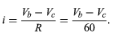(24)

Since points a and b are connected by a conductor of negligible resistance, they are at the same potential. For the same reason, c and d are at the same potential. Therefore, Vb − Vc = Va − Vd = 12 volts. The current in the circuit is given by equation (24). Thus, i = 12/60 = 0.2 ampere. The power dissipated in the resistor as heat is easily calculated using equation (22):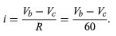(24)

P=i2R

P=i2R= (o.2)2 x 60 = 2.4 watts.

Where does the energy that is dissipated as heat in the resistor come from? It is provided by a source of electromotive force (e.g., a lead-acid battery). Within such a source, for each amount of charge dQ moved from the lower potential at d to the higher potential at a, an amount of work is done equal to dW = dQ(Va − Vd). If this work is done in a time interval dt, the power delivered by the battery is obtained by dividing dW by dt. Thus, the power delivered by the battery (in watts) is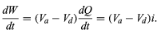Using the values i = 0.2 ampere and Va − Vd = 12 volts makes dW/dt = 2.4 watts. As expected, the power delivered by the battery is equal to the power dissipated as heat in the resistor.

Resistors in Series and Parallel:

If two resistors are connected in Figure so that all of the electric charge must traverse both resistors in succession, the equivalent resistance to the flow of current is the sum of the resistances.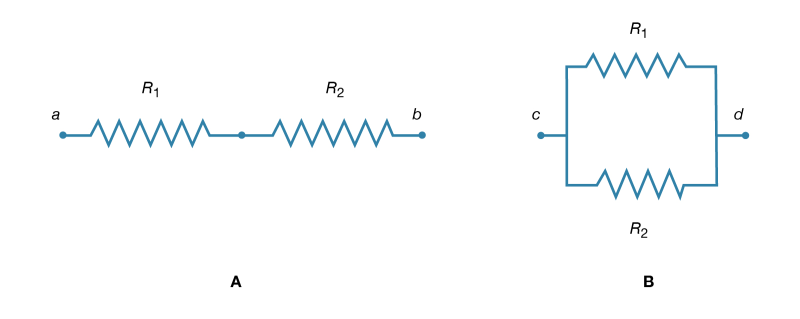Figure 16: Resistors. (A) In series. (B) In parallel.

Using R1 and R2 for the individual resistances, the resistance between a and b is given by

Rab =R1+R2      (25a)

This result can be appreciated by thinking of the two resistors as two pieces of the same type of thin wire. Connecting the wires in series as shown simply increases their length to equal the sum of their two lengths. As equation (20) indicates, the resistance is the same as that given by equation (25a). The resistances R1 and R2 can be replaced in a circuit by the equivalent resistance Rab. If R1 = 5Ω and R2 = 2Ω, then Rab = 7Ω. If two resistors are connected as shown in Figure 16 B the electric charges have alternate paths for flowing from c to d. The resistance to the flow of charge from c to d is clearly less than if either R1 or R2 were missing. Anyone who has ever had to find a way out of a crowded theatre can appreciate how much easier it is to leave a building with several exits than one with a single exit. The value of the equivalent resistance for two resistors in parallel is given by the equation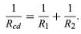(25a)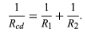(25b)

This relationship follows directly from the definition of resistance in equation (20), where 1/R is proportional to the area. If the resistors R1 and R2 are imagined to be wires of the same length and material, they would be wires with different cross-sectional areas. Connecting them in parallel is equivalent to placing them side by side, increasing the total area available for the flow of charge. Clearly, the equivalent resistance is smaller than the resistance of either resistor individually. As a numerical example, for R1 = 5Ω and R2 = 2Ω, 1/Rcd = 1/5 + 1/2 = 0.7. Therefore, Rcd= 1/0.7 = 1.43Ω. As expected, the equivalent resistance of 1.43 ohms is smaller than either 2 ohms or 5 ohms. It should be noted that both equations (25a) and (25b) are given in a form in which they can be extended easily to any number of resistances.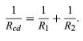(25b)

Kirchhoff’s Laws of Electric Circuits:

Two simple relationships can be used to determine the value of currents in circuits. They are useful even in rather complex situations such as circuits with multiple loops. The first relationship deals with currents at a junction of conductors. Figure 17 shows three such junctions, with the currents assumed to flow in the directions indicated.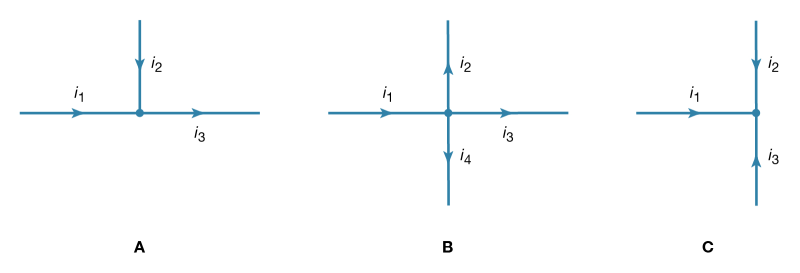Figure 17: Electric currents at a junction (see text).

Simply stated, the sum of currents entering a junction equals the sum of currents leaving that junction. This statement is commonly called Kirchhoff’s first law (after the German physicist Gustav Robert Kirchhoff, who formulated it). For Figure 17A, the sum is i1 + i2 = i3. For Figure 17B, i1 = i2 + i3 + i4. For Figure 17 C, i1 + i2 + i3 = 0. If this last equation seems puzzling because all the currents appear to flow in and none flows out, it is because of the choice of directions for the individual currents. In solving a problem, the direction chosen for the currents is arbitrary. Once the problem has been solved, some currents have a positive value, and the direction arbitrarily chosen is the one of the actual current. In the solution some currents may have a negative value, in which case the actual current flows in a direction opposite that of the arbitrary initial choice.
Kirchhoff’s second law is as follows: the sum of electromotive forces in a loop equals the sum of potential drops in the loop. When electromotive forces in a circuit are symbolized as circuit components as in Figure 15, this law can be stated quite simply: the sum of the potential differences across all the components in a closed loop equals zero. To illustrate and clarify this relation, one can consider a single circuit with two sources of electromotive forces E1 and E2, and two resistances R1 and R2, as shown in Figure 18. The direction chosen for the current i also is indicated. The letters a, b, c, and d are used to indicate certain locations around the circuit. Applying Kirchhoff’s second law to the circuit,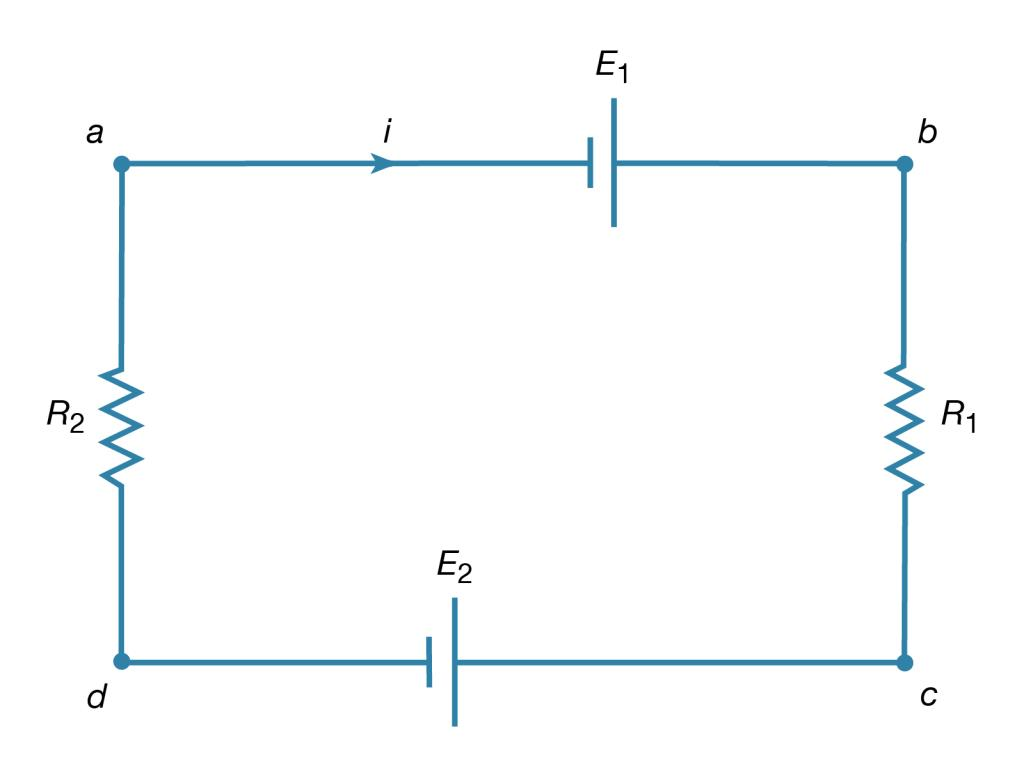Figure 18: Circuit illustrating Kirchhoff's loop equation (see text).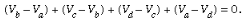(26)

Referring to the circuit in Figure 18, the potential differences maintained by the electromotive forces indicated are VbVa = E1, and VcVd = −E2. From Ohm’s law, VbVc = iR1, and VdVa = iR2. Using these four relationships in equation (26), the so-called loop equation becomes E1E2iR1iR2 = 0.
Given the values of the resistances R1 and R2 in ohms and of the electromotive forces E1 and E2in volts, the value of the current i in the circuit is obtained. If E2 in the circuit had a greater value than E1, the solution for the current i would be a negative value for i. This negative sign indicates that the current in the circuit would flow in a direction opposite the one indicated in Figure 18.
Kirchhoff’s laws can be applied to circuits with several connected loops. The same rules apply, though the algebra required becomes rather tedious as the circuits increase in complexity.

Offer running on EduRev: Apply code STAYHOME200 to get INR 200 off on our premium plan EduRev Infinity!

,

,

,

,

,

,

,

,

,

,

,

,

,

,

,

,

,

,

,

,

,

;